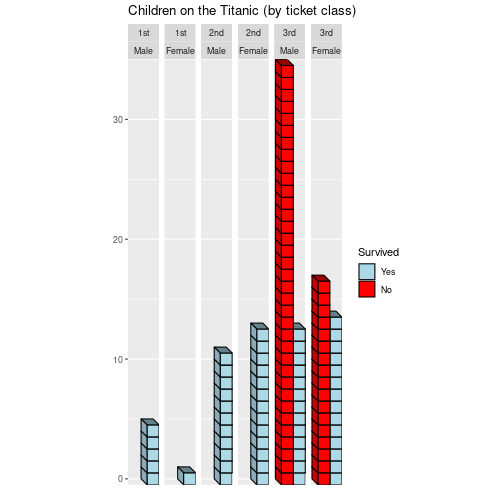# Introduction to oblicubes

## Overview

{oblicubes} is an extension for coolbutuseless’s {isocubes} that supports 3D graphics in {grid} and {ggplot2} by rendering cubes/cuboids with an oblique projection (instead of an isometric projection). As a special case we also support “primary view orthographic projections” as well. Like {isocubes} the {oblicubes} package only supports rendering non-rotated cubes (and cuboids) placed at integer coordinates. If you need to do more complex oblique projections you’ll need to use a package like {piecepackr} which supports additional shapes, supports adding art/text to their faces, rotating shapes, placing shapes at non-integer coordinates, etc. Lots of other R packages provide high quality 3D render support for other projections.

{oblicubes} {isocubes}
oblique projection, “primary view orthographic projection” isometric projection
right-handed coordinate system with z vertical left-handed coordinate system with y vertical
Use xyz_heightmap() to create x,y,z coordinates Use coord_heightmap() to create x,y,z coordinates
Use oblicubesGrob(), grid.oblicubes(), or geom_oblicubes() to render image Use isocubesGrob() to render image
Fast culling of non-visible cubes for “primary view orthographic projection”. Slower and less thorough culling of non-visible cubes for “oblique projection”. Fast culling of non-visible cubes.

Inspired by cj-holmes’s {isocuboids} package this package also supports drawing cuboids in addition to cubes. Using cuboids instead of cubes can provide significant speed advantages when rendering “height map” style images.

{oblicubes} {isocuboids}
oblique projection, “primary view orthographic projection” isometric projection
right-handed coordinate system with z vertical left-handed coordinate system with y vertical
Use xyz_heightmap(solid = FALSE) to create x,y,z coordinates Coordinates generated within image rendering functions
Use oblicuboidsGrob(), grid.oblicuboids(), or geom_oblicuboids() to render image Use cuboid_matrix(), cuboid_image() to render image

## Examples

### Different oblique projections

{oblicubes} supports different oblique projection angles:

library("grid")
library("oblicubes")
angles <- c(135, 90, 45, 180, 45, 0, -135, -90, -45)
scales <- c(0.5, 0.5, 0.5, 0.5, 0.0, 0.5, 0.5, 0.5, 0.5)
mat <- matrix(c(1, 2, 1, 2, 3, 2, 1, 2, 1), nrow = 3, ncol = 3)
coords <- xyz_heightmap(mat, col = c("red", "yellow", "green"))
vp_x <- rep(1:3/3 - 1/6, 3)
vp_y <- rep(3:1/3 - 1/6, each = 3)
for (i in 1:9) {
pushViewport(viewport(x=vp_x[i], y=vp_y[i], width=1/3, height=1/3))
grid.rect(gp = gpar(lty = "dashed"))
grid.oblicubes(coords, width = 0.15, xo = 0.25, yo = 0.15,
angle = angles[i], scale = scales[i],
gp = gpar(lwd=4))
if (i != 5)
grid.text(paste("angle =", angles[i]), y=0.92, gp = gpar(cex = 1.2))
else
grid.text(paste("scale = 0"), y=0.92, gp = gpar(cex = 1.2))
popViewport()
}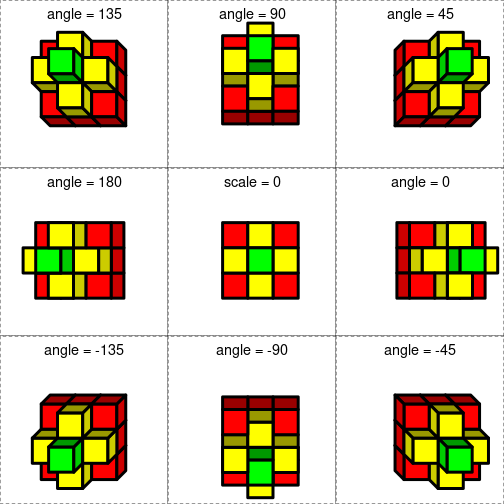### Volcano heightmap

• By default we do an oblique projection with a scale of 0.5 and an angle of 45. This is also known as a “cabinet projection”.
• Using cuboids instead of cubes can provide significant speed advantages when rendering “height map” style images.
library("grDevices")
library("ggplot2")
library("oblicubes")
data("volcano", package = "datasets")
df <- xyz_heightmap(volcano, scale = 0.3, min = 1, solid = FALSE)
g <- ggplot(df, aes(x, y, z = z, fill = raw)) +
geom_oblicuboids(light = FALSE) +
coord_fixed() +
colours=terrain.colors(256)) +
labs(x = "East (10m)", y = "North (10m)",
title = "Maungawhau (datasets::volcano)")
plot(g)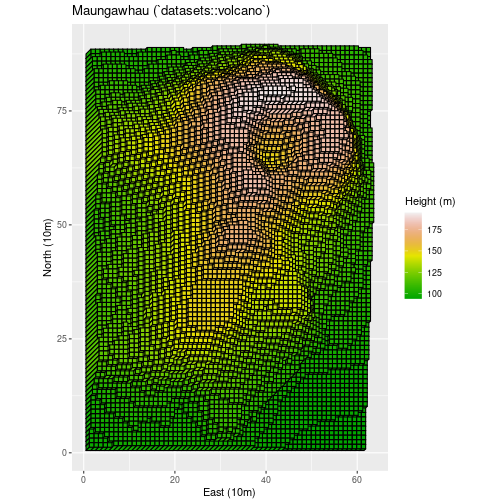• By playing around with the flipx, flipy, ground arguments in xyz_coords() it is also possible to generate views from different sides of the object.
• A scale of 0 gives you a “primary view orthographic projection”.
library("grDevices")
library("grid")
library("oblicubes")
data("volcano", package = "datasets")
mat <- 0.3 * (volcano - min(volcano)) + 1.0

grid.rect(gp=gpar(col=NA, fill="grey5"))
width <- convertWidth(unit(0.007, "snpc"), "cm")

# Top view
pushViewport(viewport(width = 0.7, height = 0.7, x = 0.65, y = 0.65))
coords <- xyz_heightmap(mat, col = terrain.colors, solid = FALSE)
grid.oblicubes(coords, scale = 0, width = width, gp = gpar(col=NA))
popViewport()

# South view
pushViewport(viewport(width = 0.7, height = 0.3, x = 0.65, y = 0.15))
coords <- xyz_heightmap(mat, col = terrain.colors, ground = "xz")
grid.oblicubes(coords, scale = 0, width = width, gp = gpar(col=NA))
popViewport()

# West view
pushViewport(viewport(width = 0.3, height = 0.7, x = 0.15, y = 0.65))
coords <- xyz_heightmap(mat, col = terrain.colors, ground = "zy")
grid.oblicubes(coords, scale = 0, width = width, gp = gpar(col=NA))
popViewport()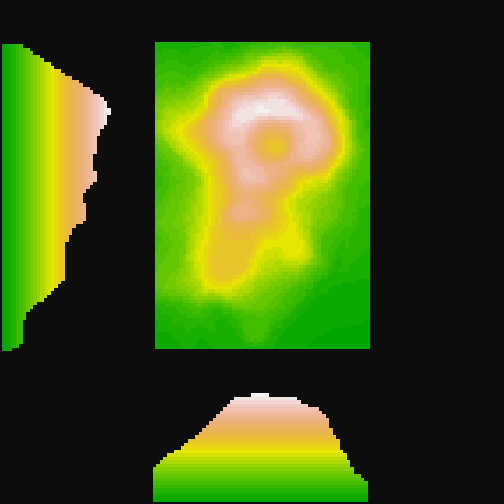### Signed distance fields

library("isocubes") # remotes::install_github("coolbutuseless/isocubes")
library("oblicubes")
sphere <- sdf_sphere() |> sdf_scale(40)
box <- sdf_box() |> sdf_scale(32)
cyl <- sdf_cyl() |> sdf_scale(16)
scene <- sdf_subtract_smooth(
sdf_intersect(box, sphere),
sdf_union(
cyl,
sdf_rotatey(cyl, pi/2),
sdf_rotatex(cyl, pi/2)
)
)
coords <- sdf_render(scene, 50)
grid.oblicubes(coords, fill = "lightgreen")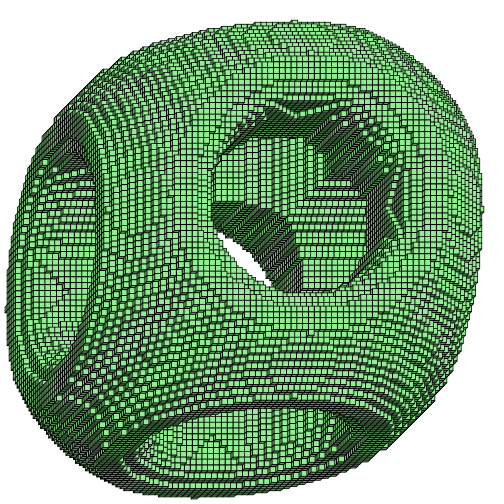### Generating fake terrain

• Here is an example generating fake terrain using “perlin noise” generated by the {ambient} package.
library("ambient")
library("oblicubes")
n <- 72
set.seed(72)
mat <- noise_perlin(c(n, n), frequency = 0.042) |>
cut(8L, labels = FALSE) |>
matrix(nrow = n, ncol = n)
coords <- xyz_heightmap(mat, col = grDevices::topo.colors, solid = FALSE)
grid.oblicuboids(coords, gp = gpar(col = NA))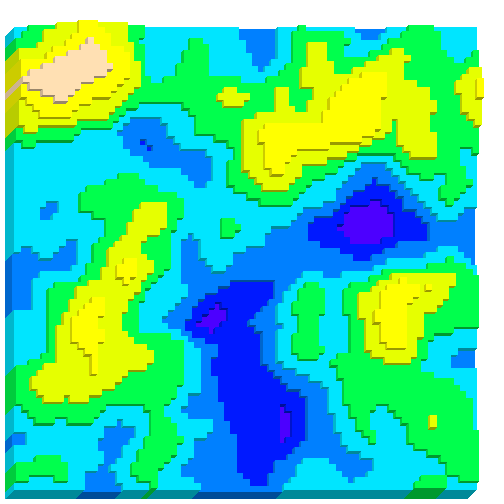### Bitmap fonts

library("bittermelon") |> suppressPackageStartupMessages()
library("oblicubes")
font_file <- system.file("fonts/spleen/spleen-8x16.hex.gz", package = "bittermelon")
bml <- as_bm_list("RSTATS", font = font)
bm <- (3 * bml) |>
bm_call(cbind) |>
bm_extend(sides = 1L, value = 1L)
col <- apply(bm + 1L, c(1, 2), function(i) {
switch(i, "white", "grey20", "lightblue", "darkblue")
})
coords <- xyz_heightmap(bm, col = col, flipy = FALSE)
grid.oblicubes(coords)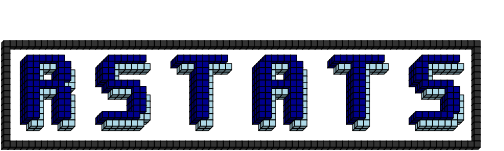### Pseudo 3D images

library("grDevices")
library("grid")
library("magick") |> suppressPackageStartupMessages()
library("oblicubes")
# Ivory model of half a human head, half a skull, Europe, undated
# Science Museum, London / CC BY 4.0
# https://wellcomecollection.org/works/z3syda8c
url <- "https://iiif.wellcomecollection.org/image/L0057080/full/760%2C/0/default.jpg"
if (!file.exists("ivory-skull.jpg"))
image_scale("20%") |>
image_crop("100x150+26+18")
col <- as.matrix(as.raster(img))
# height by luminosity
rgb2lum <- function(x) (0.2126 * x + 0.7152 * x + 0.0722 * x) / 255
mat <- col2rgb(col) |>
apply(2, rgb2lum)  |>
matrix(nrow = nrow(col), ncol = ncol(col))
df <- xyz_heightmap(mat, col, scale = 20, min = 1, solid = FALSE)
grid.newpage()
grid.rect(gp=gpar(fill="black"))
grid.raster(img, vp = viewport(x=0.25, width=0.5, just=c(0.5, 0.67)))
grid.text(paste("Ivory model of half a human head, half a skull",
"Europe, undated",
"Science Museum, London",
"Attribution 4.0 International (CC BY 4.0)",
sep = "\n"),
x=0.26, y = 0.75, gp = gpar(col = "white"))
grid.oblicuboids(df, scale=0.5, gp=gpar(col=NA),
vp = viewport(x=0.75, width=0.5))
grid.text("Pseudo 3D derivative (based on luminosity)",
x=0.76, y = 0.75, gp = gpar(col = "white"))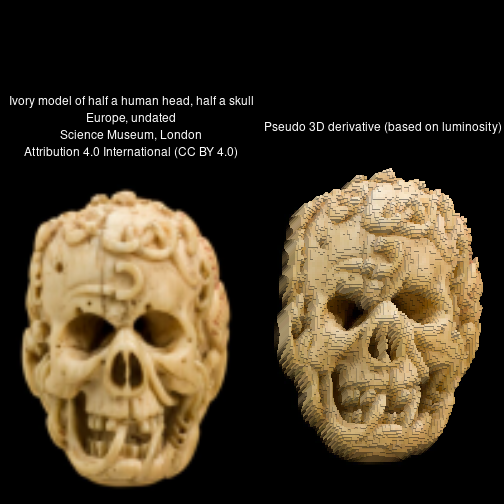### 3D bar charts

• Should you ever make a 3D bar chart? Probably not…
• If you have integer valued y-values could you use {oblicubes} to make a 3D bar chart? With some work…
• You can use yoffset and zoffset parameters to shift cubes so the top/bottom of the cubes lie on integer values (instead of the center of the cubes)
library("dplyr") |> suppressPackageStartupMessages()
library("ggplot2")
library("oblicubes")
df <- as.data.frame(datasets::Titanic) |>
filter(Age == "Child", Freq > 0) |>
group_by(Sex, Survived, Class) |>
summarize(Freq = seq.int(sum(Freq)), .groups = "drop")
ggplot(df, aes(x = Survived, y = Freq, fill = Survived)) +
facet_grid(cols = vars(Class, Sex)) +
coord_fixed() +
geom_oblicubes(yoffset = -0.5, zoffset = -0.5, angle = -45, scale = 0.7) +
scale_fill_manual(values = c("Yes" = "lightblue", "No" = "red")) +
scale_y_continuous(expand = expansion(), name = "") +
scale_x_discrete(name = "", breaks = NULL) +
labs(title = "Children on the Titanic (by ticket class)")